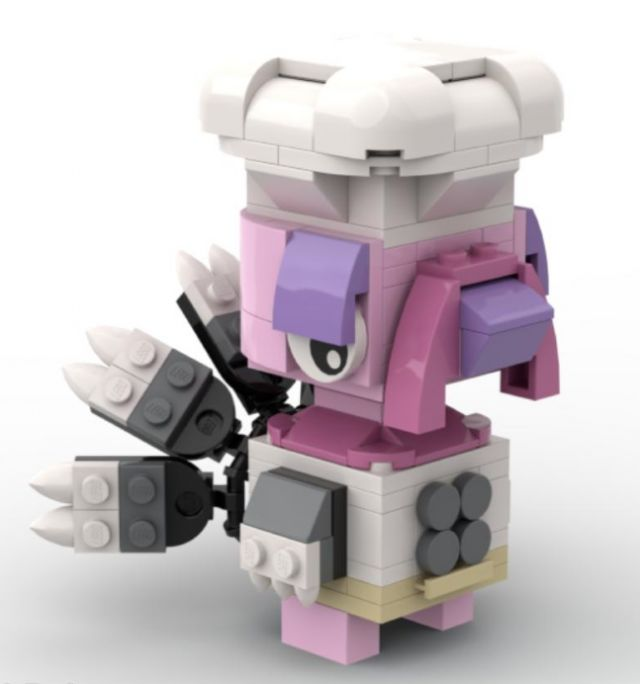-=+=- -=+=- -=+=- -=+=- -=+=- -=+=- -=+=- -=+=- -=+=- -=+=- -=+=- -=+=- -=+=- -=+=- -=+=- -=+=- -=+=- -=+=- -=+=- -=+=- -=+=- -=+=- -=+=- -=+=- -=+=- -=+=- -=+=- -=+=- -=+=- -=+=- (c) WidthPadding Industries 1987 0|152|0 -=+=- -=+=- -=+=- -=+=- -=+=- -=+=- -=+=- -=+=- -=+=- -=+=- -=+=- -=+=- -=+=- -=+=- -=+=- -=+=- -=+=- -=+=- -=+=- -=+=- -=+=- -=+=- -=+=- -=+=- -=+=- -=+=- -=+=- -=+=- -=+=- -=+=- Socoder -> Festive Threads -> Happy Thanksgiving Wed, 24 Nov 2021, 09:50 rockfordHappy Thanksgiving and Best Wishes to our American friends and family -Wed, 24 Nov 2021, 09:50 JayenkaiHappy Thanksgiving (though it's not til tomorrow!) What a lovely turkey!! -=-=- ''Load, Next List!''Wed, 24 Nov 2021, 10:07 rockfordI know it's tomorrow, but I thought I'd get it in early, as I'm on nights and might forget to post early tomorrow. CheersThe turkey is based on Franklin from Animal Crossing. In Lego formGobble Gobble Gobble! Wed, 24 Nov 2021, 21:18 KuronThank you Rockford & Jay! I was at the house today and there were 23 turkeys in the front yard. Gonna fix the dinner, the only thing I did not make is dessert. Was no room in the freezer for a frozen pie, so I opted for eight of these. May go do some work at the house while the turkey is cooking in the slow cooker. -=-=- Live Strong. Act Bold. Be Brave. Nothing's Too Hard To Do. Never Give Up. ALWAYS BELIEVE. -- Warrior Wed, 24 Nov 2021, 21:59 rockfordI like a big meal, but I doubt I could eat 23 turkeys AND pie... Have a good oneWed, 24 Nov 2021, 22:24 KuronWill be bittersweet that is for sure. Know she would want me to make a good meal and try to enjoy it, as we had already bought the food. Plan to set a place for Carolyn, plate and silverware and chair.-=-=- Live Strong. Act Bold. Be Brave. Nothing's Too Hard To Do. Never Give Up. ALWAYS BELIEVE. -- Warrior